## Sunday, January 25, 2015

### Goodies!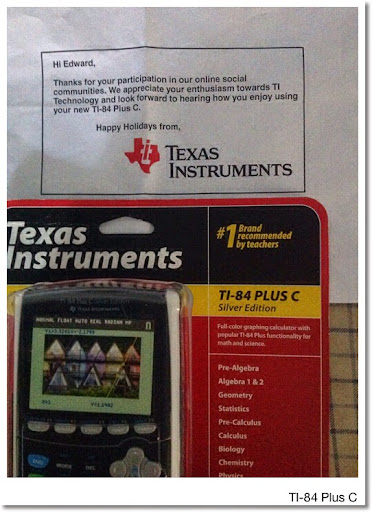Shout out to Texas Instruments for sending me a TI-84 Plus C graphing calculator last month. Thank you! (Apologizes on being late about it.)

My first graphing calculator was a TI-81, which I still have and it's working. I think I currently have four of the TI-84 family (83+ Silver, 84+, and two 84 color editions).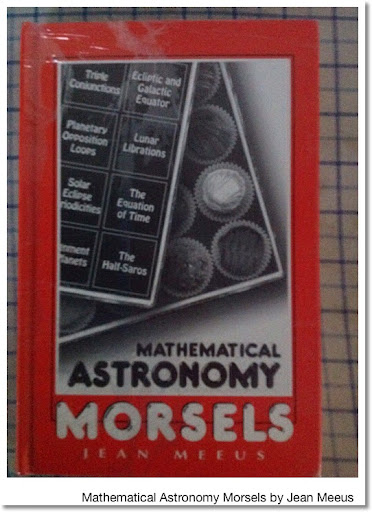My Amazon order came in last week: "Mathematical Astronomy Morsels" by Jean Meeus. It can shrink wrapped, which is nice. I found out that Meeus calculated how long the Age of Pisces was going to last (until 2597). Here, the definition of age is determined the placement of the vernal equinox.

Thanks Agena Astroproducts for quick delivery.

Just wanted to show you some of the goodies.

Eddie

This blog is property of Edward Shore. 2015

### HP Prime: Calculator Art

Programs will follow.  Enjoy!   Eddie

DRAW3DCUBE:  3D Cube - not as easy as it seems.DRAW3DCUBE
DRAW3DCYN:  My first one I did this weekend.  This looks like a tall glass of water.DRAW3DCYN
DRAW3DSPH:  Sphere.  I like the effect the HP Prime does with ARC in a FOR loop.DRAW3DSPH

DRAW3DWAVE:  I wanted something to be drawn using a sine wave.DRAW3DWAVE

DRAWSUNSET - This is probably my favorite, since I like sunsets.DRAWSUNSET

Tip:  The HP Prime screen is 318 pixels wide and 240 pixels deep.  If you want to leave room for custom menus, then the canvas is 318 pixels by 218 pixels.  The x axis increases to the right, but unlike the Cartesian plane, the y axis increases downward instead of upward.

Eddie

-------------
Programs:

DRAW3DCUBE

EXPORT DRAW3DCUBE()
BEGIN
LOCAL t,a,b,c,d,s;
RECT();
// coord
a:=159-50*COS(45°);
b:=109-50*SIN(45°);
c:=159+50*COS(45°)+1;
d:=159-50*SIN(45°);

// drawing
LINE_P(a+50*COS(45°),b-SIN(45°),a,b);
LINE_P(a,b,159,109);
LINE_P(159,109,c,b);
LINE_P(c,b,a+50*COS(45°),b-SIN(45°));

LINE_P(a,b,a,d);
LINE_P(a,d,159,159);
LINE_P(159,159,159,109);

LINE_P(159,159,c,d);
LINE_P(c,d,c,b);

FOR t FROM b TO d DO
LINE_P(a,t,159,t+50*SIN(45°),#FF0000h);
LINE_P(c,t,159,t+50*SIN(45°),#FFh);
END;

FOR t FROM 1 TO 50 DO
LINE_P(a+t*COS(45°),b-t*SIN(45°),
a+t*COS(45°),b+t*SIN(45°),#FFFF00h);
END;

FOR t FROM 50 DOWNTO 1 DO
LINE_P(c-t*COS(45°),b-t*SIN(45°),
c-t*COS(45°),b+t*SIN(45°),#FFFF00h);
END;

WAIT(0);   // I prefer WAIT(0) to FREEZE;

END;

DRAW3DCYN

EXPORT DRAW3DCYN()
BEGIN
LOCAL t;
HAngle:=0;
RECT();
// cylinder shell
FOR t FROM 0 TO 100 STEP 1 DO

ARC_P(159,70+t,20,0,π,#7DF9FFh);

ARC_P(159,70+t,20,π,2*π,#1560BDh);
END;
// sides
LINE_P(139,70,139,170,#1560BDh);
LINE_P(179,70,179,170,#1560BDh);
ARC_P(159,70,20,0,2*π,#1560BDh);
WAIT(0);

END;

DRAW3DSPH

EXPORT DRAW3DSPH()
BEGIN
// 2015-01-22
LOCAL t;
RECT();

// sphere loop
FOR t FROM 100 DOWNTO 1 DO
ARC_P(159,109,t,0,2*π,#808080h);
END;

// outside
FOR t FROM 59 TO 259 DO
PIXON_P(t,−.0025*t^2+.795*t+70.975);
END;

WAIT(0);

END;

DRAW3DWAVE

EXPORT DRAW3DWAVE()
BEGIN
LOCAL t,a,b;
RECT();
HAngle:=0;

FOR t FROM 0 TO 318 DO
a:=25*SIN(3.95168887244ᴇ−2*t-π)+100;
b:=a+25;
PIXON_P(t,a,#FFh);
PIXON_P(t,b,#FFh);

LINE_P(t,a+1,t,b-1,#87CEEBh);
LINE_P(t,b+1,t,218,#964B00h);

END;

LINE_P(0,100,0,125,#FFh);
LINE_P(318,100,318,125,#FFh);

LINE_P(0,125,0,218,#D4AF37h);
LINE_P(318,125,318,218,#D4AF37h);
LINE_P(0,218,318,218,#D4AF37h);

WAIT(0);

END;

DRAWSUNSET

EXPORT DRAWSUNSET()
BEGIN

RECT(#87CEEBh);
LOCAL t,a,b;
HAngle:=0;

// sun
FOR t FROM 50 DOWNTO 40 DO
ARC_P(159,109,t,0,2*π,#FFA500h);
END;
FOR t FROM 40 DOWNTO 0 DO
ARC_P(159,109,t,0,2*π,#FFFF00h);
END;

// horizon
LINE_P(0,108,318,108);

// ground and water
FOR t FROM 0 TO 159 DO
a:=1.855525955687ᴇ−4*t^3
-5.05717802449ᴇ−2*t^2
+3.67070350319*t+109;
LINE_P(t,a,t,109,#80h);
LINE_P(t,a+1,t,242,#964B00h);
END;

FOR t FROM 159 TO 318 DO
a:=1.23858063728ᴇ−4*t^3
-9.61799073419ᴇ−2*t^2
+23.6382711212*t
-1664.83052851;
LINE_P(t,a,t,109,#80h);
LINE_P(t,a+1,t,242,#964B00h);
END;

// lines
LINE_P(120,111,188,111,#C0C0C0h);
LINE_P(125,113,183,113,#C0C0C0h);
LINE_P(130,115,178,115,#C0C0C0h);
LINE_P(135,117,173,117,#C0C0C0h);

WAIT(0);
END;

This blog is property of Edward Shore.  2015

## Friday, January 16, 2015

### fx-5800p: Large Factorials, Length of Daylight, & Moments/Skewness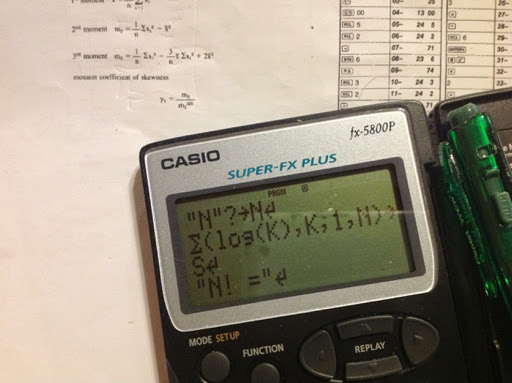fx-5800p: Large Factorials, Length of Daylight, & Moments/Skewness

Large Factorials

This allows the user to calculate n! for any positive n, even n > 69.

Program BIGFACT
"N"?→N
Σ(log(K),K,1,N) → S
"N = "
10^(Frac(S)) ◢
"* 10^( )"
Int(S)

Examples:
36! ≈ 3.719933268 * 10^ 41
134! ≈ 1.992942746 * 10^ 228

Length of Daylight

D = days after March 21. A 365 day year is assumed.

Program DAYLIGHT
"DAYS AFTER"
"MARCH 21"? → D
Deg
23.45 * sin(D*.9856) → T
"LATITUDE"
"D ● M ● S ●"? → L // for ● press [ ° ' " ]
cos⁻¹ (-tan(L) * tan(T) ) → A
2 * A / 15 → H
"HOURS="
H

Example:
D = 302 (January 17)
L = 40° N (+40°)
Result: H = 9.525548719 hours

D = 302 (January 17)
L = 40° S (-40°)
Result H = 14.47445128

Moments/Skewness

Source: HP 33 Math Pak, 1979

Formulas:

1st Moment: A = Σx ÷ n
2nd Moment: B = Σ(x^2) ÷ n - A^2
3rd Moment: C = (Σ(x^3) - 3 * A * Σ(x^2)) ÷ n + 2 * A^3
Coefficient of Skewness: D = C / B^1.5

Program MOMENTS
0 → N // Initialization
0 → U
0 → V
0 → W
Lbl 0 // Main Loop
N + 1 → N
U + X → U
V + X^2 → V
W + X^(3) → W
"NO. OF PTS."
N ◢
"ANOTHER PT.?" // are you done?
"0=NO 1=YES"
? → Y
Y ≠ 0 ⇒ Goto 0
U ÷ N → A // analysis
V ÷ N - A^2 → B
(W - 3*A*V) ÷ N + 2 * A^(3) → C
C ÷ B^(1.5) → D
"1ST MOMENT"
A ◢
"2ND MOMENT"
B ◢
"3RD MOMENT"
C ◢
"SKEWNESS"
D

Example:

Data: 1.5, 2.8, 2.9, 3.6, 4.7

Results:
1st Moment = 3.1
2nd Moment = 1.1
3rd Moment = 0.018
4th Moment = 0.0156021151

See you all next time - have a great weekend! Go have some fun!

This blog is property of Edward Shore. 2015

## Thursday, January 15, 2015

### fx-5800p: Creating a Custom Formula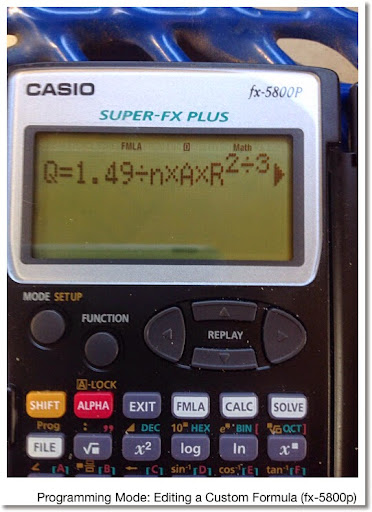fx-5800p: Creating a Custom Formula

Here is a short tutorial on how to create a custom formula for the Casio fx-5800p. The fx-5800p has a library of 128 formulas ranging in various topics from geometry to physics and engineering.

Creating an Original Formula:

1. Press [ MODE ]
2. Select 5 for Programming Mode
3. Select 1 for New
4. Give a name, no more than 12 letters or numbers. Symbols are not allowed.
5. At the File Mode screen, select 3 for Formula.
6. Type your formula. Use the y=f(x) format.
7. When you are done, press the [ EXIT ] key.

Tip: You can use lower case letters, Greek letters, and stylized letters in the formula. Press [ FUNCTION ], then 4 for ALPHA to get access to these characters.

Remember: Variables are one character length. The fx-5800p will execute implied multiplication when it executes two more consecutive variables.

1. Be in COMP Mode. (MODE, 1)
2. Press the [ FMLA ] button. Select 1 for the Original type.
3. Select your formula, press [ EXE ]
4. Use the arrow keys to enter values or scroll the formula. When you are ready to compute, press [ CALC ].

And that is about all there is to regarding creating and calculating formulas on the Casio fx-5800p.

Eddie

This blog is property of Edward Shore. 2015

## Friday, January 9, 2015

### fx-5800p: Least Squares Fitting

fx-5800p: Least Squares Fitting

Program LSQ (least squares) for the Casio fx-5800p. The program LSQ (least squares) allows us to fit a polynomial or a multiple-linear regression line given certain data and outcomes.

Variables:

Mat A = independent data matrix
Mat B = dependent data matrix, 1-column matrix
Mat C = coefficient matrix. This is the matrix LSQ solves for.
Mat D = predictive values

Calculations:

Coefficients
Mat C = (Mat A^T * Mat A)^-1 * Mat A^T * Mat B = (Mat A)^+ * Mat B

Predictive Values
Mat D = Mat A * Mat C

Prog "LSQ"
"LSQ"
"A = IND. DATA"? → Mat A
"B = DEP. DATA"? → Mat B
( Trn( Mat A ) * Mat A )^-1 * Trn( Mat A ) * Mat B → Mat C
"C = COEFS."
Mat C ◢
Mat A * Mat C → Mat D
"D = PRED."
Mat D

Examples

Multiple Linear Regression

Fit the following data to y = a * x1 + b * x2 + c:
(x1, x2, y):
(1, .6, .45)
(2, .3, .49)
(3, .2, .36)
(4, .8, .36)
(5, .6, .39)

Note that the equation has a constant term c. Set up our matrix A with three columns: one corresponding to the term a * x1, the second for the term b * x2, and a column of ones for the constant column c.

Mat A =
[[ 1, .6, 1]
[2, .3, 1]
[3, .2, 1]
[4, .8, 1]
[5, .6, 1]]

Matrix B will have the dependent values, in this case a 5 x 1 matrix.

Mat B =
[[ .45 ]
[ .49 ]
[ .36 ]
[ .38 ]
[ .39 ]]

With the matrices set up, run LSQ. You can enter the required matrices manually (with [ ALPHA ] [ ln ] for the left bracket, [ ALPHA ] [ x^ ] for the right bracket) or used pre-stored matrices. The fx-5800p has room for only six matrices (Mat A - Mat F).

Results:
Coefficients, Mat B =
[[ -0.02381395349 ]
[ 0.0162790697 ]
[ 0.4773023256 ]]

This corresponds to the equation for the line:

y = -0.02381395349 * x1 + 0.0162790697 * x2 + 0.4773023256

Predicted y values, Mat C =
[[ 0.463255813951]
[ 0.4345581395 ]
[ 0.4091162791 ]
[ 0.3950697674 ]
[ 0.368 ]]

Polynomial Regression

Fit the quadratic polynomial of form y = a*x^2 + b*x + c with data:
(-3, -2.25)
(-1, 0)
(1, -0.11)
(3, 2.23)
(5, 5.24)

We have one variable. However the equation has both x and x^2. Hence, we will set up matrix A as follows: first column has x^2 values, second has x values, and third column contains ones because it corresponds to the constant term (c).

Mat A =
[[ 9, -3, 1]
[1, -1, 0]
[1, 1, -0.11]
[9, 3, 1]
[25, 5, 1]]

Again, matrix B has the dependent values:

Mat B =
[[ -2.24 ]
[ 0 ]
[ -0.11 ]
[ 2.23 ]
[ 5.24 ]]

Results:

Mat C =
[[ 0.07125 ]
[ 0.717 ]
[ -0.33425 ]]

which corresponds to: y = 0.07125 * x^2 + 0.717 * x - 0.33425

Mat D =
[[ -1.844 ]
[ -0.98 ]
[ 0.454 ]
[ 2.458 ]
[ 5.032 ]]

Even though the least-squares method isn't perfect, it is super powerful. Until next time, have a great day, stay healthy, and stave off any viruses because they have gone crazy this winter!

Eddie

Source: http://en.m.wikipedia.org/wiki/Regression_analysis

This blog is property of Edward Shore. 2015

## Monday, January 5, 2015

### fx-5800p: Runge-Kutta 4th Order Method - Differential Equations

Runge-Kutta 4th Order Method - Differential Equations

The program RK4 estimates solutions to the differential equation y dy/dx = f(x,y) given the initial condition (x_n, y_n). With step size h, the point (x_n+1, y_n+1) is calculated by:

x_n+1 = x_n + h
y_n+1 = y_n + (k1 + 2*k2 + 2*k3 + k4)/6

Where:

k1 = h * f(x_n, y_n)
k2 = h * f(x_n + h/2, y_n + k1/2)
k3 = h * f(x_n + h/2, y_n + k2/2)
k4 = h * f(x_n + h, y_n + k3)

The program RK4 uses a subroutine FXY. The program FXY is where the y'(x) is stored in terms of X and Y. The result is stored in the variable Z.

Variables used in RK4 and FXY:
A = x_n
B = y_n
C = x_n+1
D = y_n+1
H = step
K = k1
L = k2
M = k3
N = k4
E, X, Y, and Z are also used

Remember for the fx-5800p, all variables (A-Z, and Z[#]s) are global, meaning they will carry over from programs and subroutines.

Anything following the double slash (//) is a comment.

Once the first point (x_n+1, y_n+1) is calculated, RK4 will ask if you want the next point (x_n+2, y_n+2) calculated. Enter 1 at the prompt for "Yes".

Program FXY
type f(X,Y) here → Z

// A RETURN command is implicit.

Program RX4
"INITIAL COND."
"X0"?→ A
"Y0"?→ B
"STEP"?→ H
Lbl 0 // main routine
A → X // k1
B → Y
Prog "FXY"
H * Z → K
A + H ÷ 2 → X // k2
B + K ÷ 2 → Y
Prog "FXY"
H * Z → L
B + L ÷ 2 → Y // k3
Prog "FXY"
H * Z → M
A + H → X // k4
B + M → Y
Prog "FXY"
H * Z → N
X → C // x_n+1
B + (K + 2*L + 2*M + N) ÷ 6 → D // y_n+1
"RESULT"
"X1 = "
C ◢
"Y1 = "
D ◢
"NEXT POINT?"
"1 = YES 0 = NO"
? → E
E ≠ 1 ⇒ Goto E
C → A
D → B
Goto 0
Lbl E
"DONE"

Example: y'(x) = y - x with the initial condition (0,2).
Find y when x = 0.1 and x = 0.2, respectively.
In this case, our step is h = 0.1.

Program FXY will have this:
Y - X → Z

Running RK4 gives these results:
x = 0.1, y = 2.205170833
x = 0.2, y = 2.421402571

Eddie

This blog is property of Edward Shore. 2015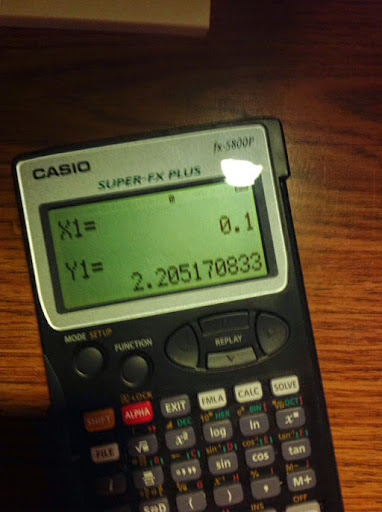## Sunday, January 4, 2015

### fx-5800p: Bisection Method

fx-5800p: Bisection Method

The program BISECT finds a root for f(X) given an initial open interval of (A, B). I have the tolerance set to 10^-9. To prevent calculations from going "forever", I set the maximum number of iterations to 150. You can adjust these factors in the program as desired.

The first point to be tested is (A+B)/2. If this is a root, the program stops and it will return this root as a result.

The program BISECT calls on a subroutine program FX. The function f(X) is stored in FX.

While programming, use the Prog to call subroutines. The syntax is:

Prog "name of subroutine in quotes"

Press [SHIFT] [FILE].

Remember for the fx-5800p, all variables (A-Z, and Z[#]s) are global, meaning they will carry over from programs and subroutines.

Anything following the double slash (//) is a comment.

Program FX
insert function of X here → Y
//store result in Y

// A Return command is implied.

Program BISECT
1 → I // I = iteration count
"A"? → A
"B"? → B
A → X
Prog "FX"
Y → C
B → X
Prog "FX"
Y → D
While Abs(A-B) > 10^(-9) // tolerance setting
(A + B) ÷ 2 → X
Prog "FX"
If Y = 0
Then
Break
IfEnd
If Y*C > 0
Then
X → A
Y → C
Else
X → B
Y → D
IfEnd
I + 1 → I
If I > 150 // iteration limit
"ITER. EXCEEDED" ◢
Stop
IfEnd
WhileEnd // finish the loop
"ITERATIONS:" ◢ // optional
I ◢ // optional
"ROOT=" ◢ // result
X

Example:

f(X) = X^(3)-2X+1, for the intervals (-2,0), (0,2), and (0,1).

Program FX would look like this:

Program FX
X^3 - 2*X + 1 → Y

Run BISECT.

For (-2,0), results are:
ITERATIONS: 32
ROOT = -1.618033989

For (0,2), results are:
ITERATIONS: 1
ROOT = 1

For (0,1), results are:
ITERATIONS: 31
ROOT = 0.6180339893

This blog is property of Edward Shore. 2015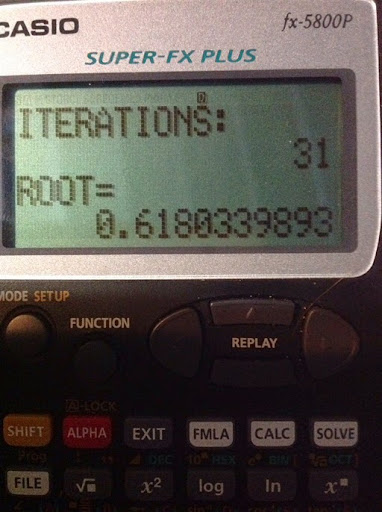### New HP Prime Software Version Released (6975)

HP Prime Software Version 6975

Rev. 6975, date 12/3/2014.

The above link provides files for the calculator, connectivity kit, and emulator.

--------------------------------------------------------------------------------------------------------------------------

The Museum of HP Calculators

Here a great place to discuss calculators, specifically HP calculators.  It is a really great place for HP calculator owners.  The link to the forum is here:  http://www.hpmuseum.org/forum/.

--------------------------------------------------------------------------------------------------------------------------

DISCLAIMER: I am not an employee of Hewlett Packard.

Have fun,

Eddie

## Thursday, January 1, 2015

### TI-84+: Windchill Factor, Boiling Point at Various Elevations, Velocity of Seismic Waves

Happy New Year!  Time flies when we are having fun - we are in 2015 - 15 years into the 21st century.  Here's to making 2015 happy and prosperous for all!

Without further ado, here are the first programs for 2015!

Wind-Chill Factor

The equation, provided by NOA 2011, to determine wind chill is:

W = 35.74 + .6215*T – 35.75*V^.16 + .4275*T*V^.16

Where:
T = air temperature (°F)
V = speed of the wind (mph)

Program WINDCHIL
: Input “AIR TEMP IN °F:”, T
: Input “WIND SPEED (MPH):”, V
: 35.75+.6215*T-35.75*V^.16+.4275*T*V^.16→W
: Disp “WIND CHILL FACTOR:”, W

Example 1:
V = 25 mph, T = 50°F.  Result:  W = 42.75596219
V = 40 mph, T = 20°F.  Result:  W = -.9093161248

Source:  Thomas J. Glover.  “Pocket Ref: 4th Ed” – 2012.  Sequoia Publishing, Inc.:  Littleton, CO

Boiling Point at Various Elevations

Using data   from Pocket Ref, the boiling point of water at certain elevations can be estimated by the following equation:

Y » 211.99262 – (1.94802*10^-3)*X + (7.17273*10^-9)*X^2

X = elevation in feet
Y = boiling point of water in °F

Program BOILH20:
: Input “ELEV. IN FEET:”, X
: 211.99262-(1.94802E-3)*X+(7.17273E-9)*X^2→Y
: Disp “APPROX BAIL PT. (°F):”, Y

Examples:
 X = elevation (feet) Y = boiling point (°F) 250 211.5060633 1000 210.0517727 2750 206.6898088

Source:  Thomas J. Glover.  “Pocket Ref: 4th Ed” – 2012.  Sequoia Publishing, Inc.:  Littleton, CO

Velocity of P-Waves and S-Waves

In measuring seismic waves (earthquakes) of p-waves (primary waves) and s-waves (secondary waves), the following equations can be used:

(I)     First Lamé Parameter:
λ = (v*E)/((1+v)*(1-2*v))

Where:
v = ratio of soil.  The following parameters are stated from the Foundation Engineering Handbook:

 Type of Soil v = Clay, above the water table 0.4 Saturated Clay, below the water table 0.5 Wet Sand 0.35 Dry Sand or Rock 0.25

E = elasticity of the soil, measured in thousand-feet per pound

(II)   Shear Modulus (modulus of rigidity)
‘μ = E/(1 + v)

(III) Velocity of the P-Wave (feet/second)
P = √((λ+2μ)*g*1000/w)

w = specific soil weight, in pounds per cubic foot
g = Earth’s gravity constant,  g = 32.1740486 ft/s^2

(IV) Velocity of the S-Wave (feet/second)
S = √(μ*1000*g/w)

Program SEISMIC
: Disp “ELASTICITY (1000 LB/FT)”
: Prompt E
: Disp “SPEC. SOIL WEIGHT”, “(LB/FT^3)”
: Prompt W
: Menu(“SOIL TYPE”, “CLAY”, 1, “BELOW H20 TABLE”, 2, “WET SAND”, 3,
“DRY SAND/ROCK”, 4)
: Lbl 1
: .4→V
: Goto 5
: Lbl 2
: .5→V
: Goto 5
: Lbl 3
: .35→V
: Goto 5
: Lbl 4
: .25→V
: Goto 5
: Lbl 5
: (EV)/((1+V)(1-2V))→L
: E/(2(1+V))→M
: √((L+2M)*32174.0486/W)→P
: √(M*32174.0486/W)→S
: Disp “P-WAVE (FT/S)”,P
: Disp “S-WAVE (FT/S)”,S

Example:
Input:
E = 3000 pounds per square foot
w = 120 pounds per cubic foot
Type of soil: Clay (v=.4)
Output:
P-Wave Velocity » 1312.863186 ft/s
S-Wave Velocity » 535.9741515 ft/s

Sources:

Hsai-Yang Fang.  “Foundation Engineering Handbook” Springer Science & Business Media.  1990.

Indrahil Goswami, Ph.D. P.E. “Civil Engineering PE Breadth and Depth, Exam Guide.  2nd Edition” McGraw Hill. 2014

----

This blog is property of Edward Shore. 2015.

### HP Prime: Linear Exponential Combination Fit

HP Prime:  Linear Exponential Combination Fit The program LINEXPREG attempts to fit bivariate data to the curve: y = a + b * x + c * e^x Th...